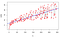Reducible vs irreducible error

Suppose that we want to predict a value Y based upon a set X = (X1, X2, …, Xp) of variables. For the predictions to have any chance of being good predictions, X needs to contain the core set of variables that drive the behavior of Y. But there will almost always be lesser variables, not included in X, that nonetheless exert some minor influence on Y. We capture the situation as follows:

Here, f is the function describing the relationship between X and Y, and ɛ is an error term that accounts for all the unmeasured influences on Y. We assume that ɛ is independent of X and has mean 0.

Usually we don’t know f exactly, so we use statistical methods (such as linear regression) to estimate f. We use to denote this estimate. This allows us to predict Y from X using the following:

Our predictions will generally be imperfect: there will be some nonzero difference between the predicted and observed values. This difference is called prediction error.The blue line is the prediction, and the red points are the observed values. The gray segments represent the prediction error.

To minimize prediction error, we need to understand its source. Broadly speaking there are two: reducible error and irreducible error.

Reducible error is the error arising from the mismatch between and f. f is the true relationship between X and Y, but we can’t see f directly— we can only estimate it. We can reduce the gap between our estimate and the true function by applying improved methods.

Irreducible error arises from the fact that X doesn’t completely determine Y. That is, there are variables outside of X — and independent of X— that still have some small effect on Y. The only way to improve prediction error related to irreducible error is to identify these outside influences and incorporate them as predictors.

To learn more about how we can further decompose reducible error, see my post The bias-variance tradeoff.

Creating the chart

To create the chart above, first download the Advertising.csv dataset from the ISLR web site. Then in R do the following:

# Get observations
# Generate predictions
tv <- as.numeric(unlist(sales.data["TV"]))
sales <- as.numeric(unlist(sales.data["sales"]))
lm.fit <- lm(sales ~ tv)
pred <- predict(lm.fit)
# Plot them
plot(tv, sales, type="n")
segments(tv, sales, tv, pred, col="darkgray")
abline(lm.fit, col="blue", lwd=2)
points(tv, sales, col="red", pch=20, lwd=2)

Written by

Willie Wheeler

Willie Wheeler's personal blog

Welcome to a place where words matter. On Medium, smart voices and original ideas take center stage - with no ads in sight. Watch
Follow all the topics you care about, and we’ll deliver the best stories for you to your homepage and inbox. Explore
Get unlimited access to the best stories on Medium — and support writers while you’re at it. Just \$5/month. Upgrade